Courses

# Test: Analog Electronics - 2

## 25 Questions MCQ Test Analog Electronics | Test: Analog Electronics - 2

Description
This mock test of Test: Analog Electronics - 2 for Electrical Engineering (EE) helps you for every Electrical Engineering (EE) entrance exam. This contains 25 Multiple Choice Questions for Electrical Engineering (EE) Test: Analog Electronics - 2 (mcq) to study with solutions a complete question bank. The solved questions answers in this Test: Analog Electronics - 2 quiz give you a good mix of easy questions and tough questions. Electrical Engineering (EE) students definitely take this Test: Analog Electronics - 2 exercise for a better result in the exam. You can find other Test: Analog Electronics - 2 extra questions, long questions & short questions for Electrical Engineering (EE) on EduRev as well by searching above.
QUESTION: 1

### Figure is a 24 Vr stabilized power supply. The zener is 24 V, 600 mW. The minimum zener current is 10 mA. Proper values R and maximum load current are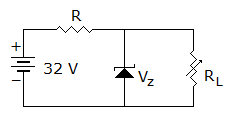Solution:

Max. zener current =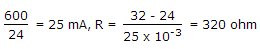.

Maximum load current = 25 - 10 = 15 mA.

QUESTION: 2

### The open loop gain of an amplifier is 200. If negative feedback with β = 0.2 is used, the closed loop gain will be

Solution:

Closed loop gain =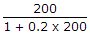.

QUESTION: 3

### In figure as the load resistance is changed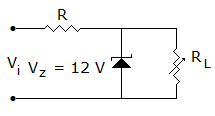Solution:

Output voltage = 12 V,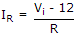.

QUESTION: 4

In a class C power amplifier the input signal has a frequency of 250 kHz. If the collector current pulses are 0.1 μs wide, the duty cycle of current waveform is

Solution: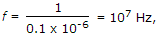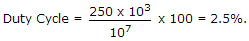QUESTION: 5

In figure, voltage across R2 = + 10 V. If VBE = 0.7 V and VE = 0.7 V and RE = 10 kΩ, current through RE is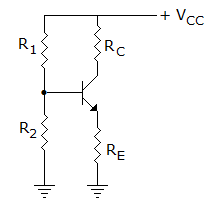Solution: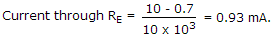QUESTION: 6

A 12 kHz pulse wave-form is amplified by a circuit having an Upper cut-off frequency of 1 MHz. The minimum input pulse width that can be accurately reproduced is

Solution: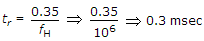tr = 10% Pω =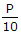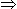minimum Power(P) = 10 tr10 x 0.35 μ sec = 3.5 μ sec.

Note: The I/P Pulse will be severely distorted if the rise time is more than 10% of Pulse width.

QUESTION: 7

Feedback factor may be less or more than 1.

Solution:

Feedback factor is much less than 1.

QUESTION: 8

An RC oscillator uses

Solution:

One RC combination can give a phase shift of less them 90º. Therefore 3 RC combinations are required for 180º phase shift.

QUESTION: 9

The main advantage of CMOS circuit is

Solution:

Low power consumption is a big advantage in digital circuits.

QUESTION: 10

Ac signals are given to both inverting and non-inverting terminals of an op-amp. When will the output maximum

Solution:

For non-inverting terminal input, output is in phase with input. For inverting terminal input, output is 180º out of phase with input. If inputs have 180º phase difference, outputs will be in phase and additive.

QUESTION: 11

An ideal op-amp has zero slew rate.

Solution:

An ideal op-amp has infinitely fast slew rate.

QUESTION: 12

In a bridge rectifier circuit the rms value of input ac voltage is 10 V. The PIV across each diode is

Solution:

PIV is 2 x 10 = 14.14 V.

QUESTION: 13

In a half wave diode rectifier circuit the current flows in the load circuit for

Solution:

Current flows during positive half cycle.

QUESTION: 14

In a push pull circuit

Solution:

Since each transistor conducts for 180º. Therefore high efficiency but low distortion.

QUESTION: 15

A transistor with a = 0.9 and ICBO = 10 μA is biased so that IBQ = 90 μA. Then IEQ will be

Solution:

IEQ = ICQ + IBQ = 910 μA + 90 μA990 μA.

QUESTION: 16

In figure, transistor βdc = 100 and LED voltage when it is conducting is 2 V. Then the base current which saturates the transistor is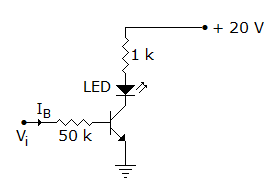Solution: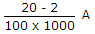.

QUESTION: 17

In figure the dc emitter current of each transistor is about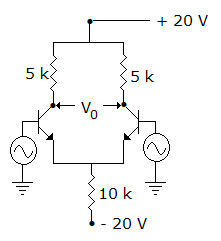Solution:

Total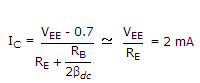. It divides equally between the two transistors.

QUESTION: 18

The input voltage for starting oscillations in an oscillator is caused by

Solution:

The amplifier in the oscillator amplifies the noise voltages. However phase shift around the closed loop is zero at only one frequency. Therefore, only this frequency appears at output.

QUESTION: 19

The current flowing in a certain P-N junction at room temperature is 2 x 10-7 Amp. When a large reverse biased voltage is applied. Calculate the current flowing when 0.1 volts is applied.

Solution: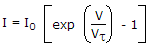QUESTION: 20

In following figure find VDSQ by assuming gate current is negligible for the p-channel JFET. (if IDQ = - 6 mA, RS = 0, VDD = -18 V, RD = 2 kΩ, IDSS = - 10 mA, IPO = - 3 V)

Solution:

By Appling KVL around drain source loop.

VDSQ = -18 - (-6). 2 x 103 = - 18 + 12 k x 10-3-6 V.

QUESTION: 21

Which of the following power amplifiers has highest efficiency?

Solution:

Class C has more efficiency than all other classes.

QUESTION: 22

A full wave rectifier using centre tapped transformer and a bridge rectifier use similar diodes and have equal no load output voltage. Under equal load conditions

Solution:

Since two diodes are in series bridge rectifier, voltage drop is more.

QUESTION: 23

Assume that op-amp in figure is ideal. If input Vi is triangular, the output V0 will be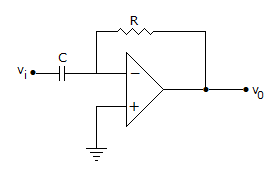Solution:

It is a differentiating amplifier.

QUESTION: 24

In an amplifier with a gain of - 1000 and feedback factor β = - 0.1, the change in gain is 20% due to temperature. The change in gain for feedback amplifier will be

Solution: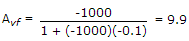. When gain changes,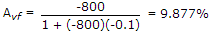age change in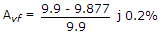QUESTION: 25

In the diode circuit of figure the diodes are ideal. The average current through ammeter is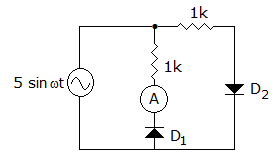Solution: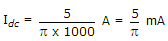.